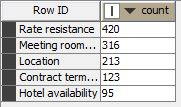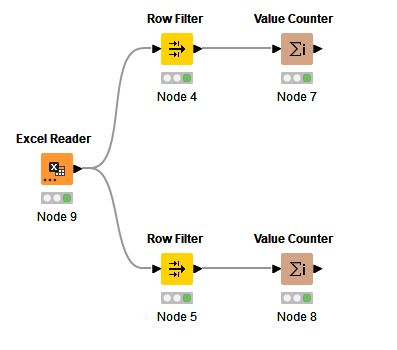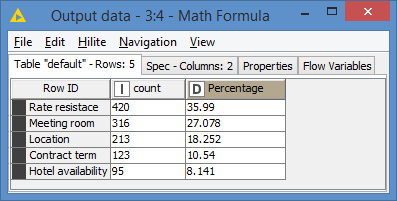# Calculate percentage after Value counterHello there,

How can I calculate the percentage of each row ID? What node should I be using next?

For example: Hotel availability % = 95/(420+316+213+123+95) *100%

My workflow is relatively simple as follows:Sincerely,

Hi @doan_lai and welcome to the Knime Community.

You can do this percentage via a Math Formula node.

EDIT: Here’s a bit more details:

`\$count\$ * 100 / COL_SUM(\$count\$)`

Results:3 Likes

Welcome to the forum @doan_lai.

If you want to keep this approach, then as @bruno29a pointed out, you can use the Math Formula node with the expression: `\$count\$/COL_SUM(\$count\$)*100`

I’d recommend looking at the GroupBy node, as you may be able to combine all these steps into a single node. You’d group by the identifying text column and aggregate on another column using Percent.

3 Likes

Thank you all kindly! I have been able to accomplish what I set out to do.

Now, I’ve been trying to connect it with Bar Chart to express the data visually. However, it cannot be done because “No column in spec compatible to “NominalValue””. I think I need to have another step to convert the output data in Math Formula node into Nominal Value.

How may I express the Percentage into Barchart from Math Formula node? Thank you in advance!

I don’t think that the percentage column is the problem.

I think that the problem is that you’re using strings as RowIDs to capture the description of each row, instead of using a separate dedicated column for this purpose.

The Bar Chart node needs a category column, and you don’t seem to have one. Therefore, you’ll need to make one. You can try using the RowID node to create a category column from the existing RowIDs.

Using the GroupBy node to calculate the percentages might’ve allowed you to avoid this issue.

1 Like

This topic was automatically closed 90 days after the last reply. New replies are no longer allowed.